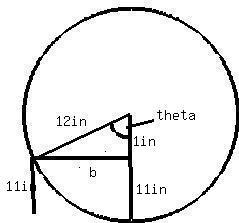Question# As you stop your car at a traffic light, a pebble becomes wedged between the tire treatds. When you start off, the distance of the pebble from the pav

Solid Geometry
ANSWEREDAs you stop your car at a traffic light, a pebble becomes wedged between the tire treatds. When you start off, the distance of the pebble from the pavement varies sinusoidally with the distance you have traveled. Assume the diameter of the wheel is 24 inches. Find the first two distances you have traveled when the pebble in the tire tread is 11 inches above the pavement.2020-12-29This answer may be more complicated than needed but here itis. First we need to set up a right triangle. The hypot. is 12in because that is the radius. second we know that one leg is 1 in use pyth. theor. to solve for third leg
$$\displaystyle{b}=\sqrt{{{12}^{{2}}-{1}^{{2}}}}=\sqrt{{{143}}}$$
now we can use tha law of sines to figure out theta
$$\displaystyle{\frac{{{\sin{{\left(\theta\right)}}}}}{{\sqrt{{{143}}}}}}={\frac{{{\sin{{\left({90}\right)}}}}}{{{12}}}}$$
So $$\displaystyle\theta={{\sin}^{{-{1}}}{\left({\sin{{\left({90}\right)}}}\cdot{\frac{{\sqrt{{{143}}}}}{{{12}}}}\right)}}$$
$$\displaystyle\theta$$ is approximately $$\displaystyle{85.2}^{\circ}$$
Now we know theta is the central angle so we canb plug itinto the formula
$$\displaystyle\text{Arc Length}={\left({\frac{{\text{central angle}}}{{{360}^{\circ}}}}\right)}\cdot{2}\pi{r}$$
$$\displaystyle={\left({\frac{{{85.2}}}{{{360}^{\circ}}}}\right)}\cdot{2}\pi{12}$$
$$\displaystyle={17.844}$$
Second distance
$$\displaystyle{360}-{85.2}={274.8}$$
$$\displaystyle{\left({\frac{{{274.8}}}{{{360}}}}\right)}\cdot{2}\pi{12}={57.55}$$
This is the actual arc length.